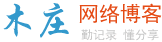# go语言math包中有哪些常用方法

go语言math包中的常用方法有：1、取绝对值方法Abs()；2、幂次方方法Pow()；3、开平方方法Sqrt()；4、开立方方法Cbrt()；5、向上取整方法Ceil()；6、向下取整方法Floor()。（学习视频分享：编程视频）

math包中常用的方法如下所示：

 12345678910111213141516171819202122232425262728293031323334353637383940414243444546474849505152535455565758596061626364 `package main` `import (``    ``"fmt"``    ``"math"``)` `func main() {``    ``/*``        ``取绝对值,函数签名如下:``            ``func Abs(x float64) float64``    ``*/``    ``fmt.Printf(``"[-3.14]的绝对值为:[%.2f]\n"``, math.``Abs``(-3.14))` `    ``/*``        ``取x的y次方，函数签名如下:``            ``func Pow(x, y float64) float64``    ``*/``    ``fmt.Printf(``"的16次方为:[%.f]\n"``, math.Pow(2, 16))` `    ``/*``        ``取余数，函数签名如下:``            ``func Pow10(n int) float64``    ``*/``    ``fmt.Printf(``"10的次方为:[%.f]\n"``, math.Pow10(3))` `    ``/*``        ``取x的开平方，函数签名如下:``            ``func Sqrt(x float64) float64``    ``*/``    ``fmt.Printf(``"的开平方为:[%.f]\n"``, math.Sqrt(64))` `    ``/*``        ``取x的开立方，函数签名如下:``            ``func Cbrt(x float64) float64``    ``*/``    ``fmt.Printf(``"的开立方为:[%.f]\n"``, math.Cbrt(27))` `    ``/*``        ``向上取整，函数签名如下:``            ``func Ceil(x float64) float64``    ``*/``    ``fmt.Printf(``"[3.14]向上取整为:[%.f]\n"``, math.``Ceil``(3.14))` `    ``/*``        ``向下取整，函数签名如下:``            ``func Floor(x float64) float64``    ``*/``    ``fmt.Printf(``"[8.75]向下取整为:[%.f]\n"``, math.``Floor``(8.75))` `    ``/*``        ``取余数，函数签名如下:``            ``func Floor(x float64) float64``    ``*/``    ``fmt.Printf(``"[10/3]的余数为:[%.f]\n"``, math.Mod(10, 3))` `    ``/*``        ``分别取整数和小数部分,函数签名如下:``            ``func Modf(f float64) (int float64, frac float64)``    ``*/``    ``Integer, Decimal := math.Modf(3.14159265358979)``    ``fmt.Printf(``"[3.14159265358979]的整数部分为:[%.f],小数部分为:[%.14f]\n"``, Integer, Decimal)` `}`### 评论

• 欢迎访问木庄网络博客
• 可复制：代码框内的文字。
• 方法：Ctrl+C。